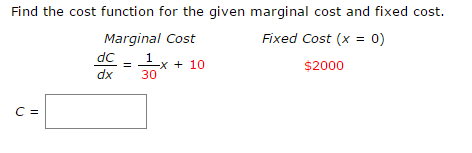## Find the cost function for the given marginal cost and fixed cost.

Find the cost function for the given marginal cost and fixed cost.

Marginal Cost
$$\frac{d}{\:dx}\:=\:\frac{1}{30}\:x\:+\:10$$

Fixed Cost
$$x\:=\:0$$
$$2000$$

$$C = ?$$

_______________________________________________________________Solved